Home » Here’s All you Need to Know About Encoding Categorical Data (with Python code)

# Here’s All you Need to Know About Encoding Categorical Data (with Python code)

## Overview

• Understand what is Categorical Data Encoding
• Learn different encoding techniques and when to use them

## Introduction

The performance of a machine learning model not only depends on the model and the hyperparameters but also on how we process and feed different types of variables to the model. Since most machine learning models only accept numerical variables, preprocessing the categorical variables becomes a necessary step. We need to convert these categorical variables to numbers such that the model is able to understand and extract valuable information.

##A typical data scientist spends 70 – 80% of his time cleaning and preparing the data. And converting categorical data is an unavoidable activity. It not only elevates the model quality but also helps in better feature engineering. Now the question is, how do we proceed? Which categorical data encoding method should we use?

In this article, I will be explaining various types of categorical data encoding methods with implementation in Python.

In case you want to learn concepts of data science in video format, check out our course- Introduction to Data Science

## Table of content

• What is Categorical Data?
• Label Encoding or Ordinal Encoding
• One hot Encoding
• Dummy Encoding
• Effect Encoding
• Binary Encoding
• BaseN Encoding
• Hash Encoding
• Target Encoding

## What is categorical data?

Since we are going to be working on categorical variables in this article, here is a quick refresher on the same with a couple of examples. Categorical variables are usually represented as ‘strings’ or ‘categories’ and are finite in number. Here are a few examples:

1. The city where a person lives: Delhi, Mumbai, Ahmedabad, Bangalore, etc.
2. The department a person works in: Finance, Human resources, IT, Production.
3. The highest degree a person has: High school, Diploma, Bachelors, Masters, PhD.
4. The grades of a student:  A+, A, B+, B, B- etc.

In the above examples, the variables only have definite possible values. Further, we can see there are two kinds of categorical data-

• Ordinal Data: The categories have an inherent order
• Nominal Data: The categories do not have an inherent order

In Ordinal data, while encoding, one should retain the information regarding the order in which the category is provided. Like in the above example the highest degree a person possesses, gives vital information about his qualification. The degree is an important feature to decide whether a person is suitable for a post or not.

While encoding Nominal data, we have to consider the presence or absence of a feature. In such a case, no notion of order is present. For example, the city a person lives in. For the data, it is important to retain where a person lives. Here, We do not have any order or sequence. It is equal if a person lives in Delhi or Bangalore.

For encoding categorical data, we have a python package category_encoders. The following code helps you install easily.

`pip install category_encoders`

## Label Encoding or Ordinal Encoding

We use this categorical data encoding technique when the categorical feature is ordinal. In this case, retaining the order is important. Hence encoding should reflect the sequence.

In Label encoding, each label is converted into an integer value. We will create a variable that contains the categories representing the education qualification of a person.

```import category_encoders as ce
import pandas as pd
train_df=pd.DataFrame({'Degree':['High school','Masters','Diploma','Bachelors','Bachelors','Masters','Phd','High school','High school']})

# create object of Ordinalencoding
encoder= ce.OrdinalEncoder(cols=['Degree'],return_df=True,
mapping=[{'col':'Degree',
'mapping':{'None':0,'High school':1,'Diploma':2,'Bachelors':3,'Masters':4,'phd':5}}])

#Original data
train_df
``````#fit and transform train data
df_train_transformed = encoder.fit_transform(train_df)```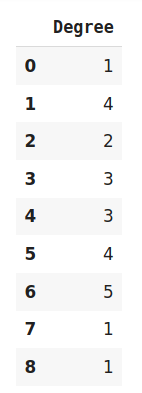## One Hot Encoding

We use this categorical data encoding technique when the features are nominal(do not have any order). In one hot encoding, for each level of a categorical feature, we create a new variable. Each category is mapped with a binary variable containing either 0 or 1. Here, 0 represents the absence, and 1 represents the presence of that category.

These newly created binary features are known as Dummy variables. The number of dummy variables depends on the levels present in the categorical variable. This might sound complicated. Let us take an example to understand this better. Suppose we have a dataset with a category animal, having different animals like Dog, Cat, Sheep, Cow, Lion. Now we have to one-hot encode this data.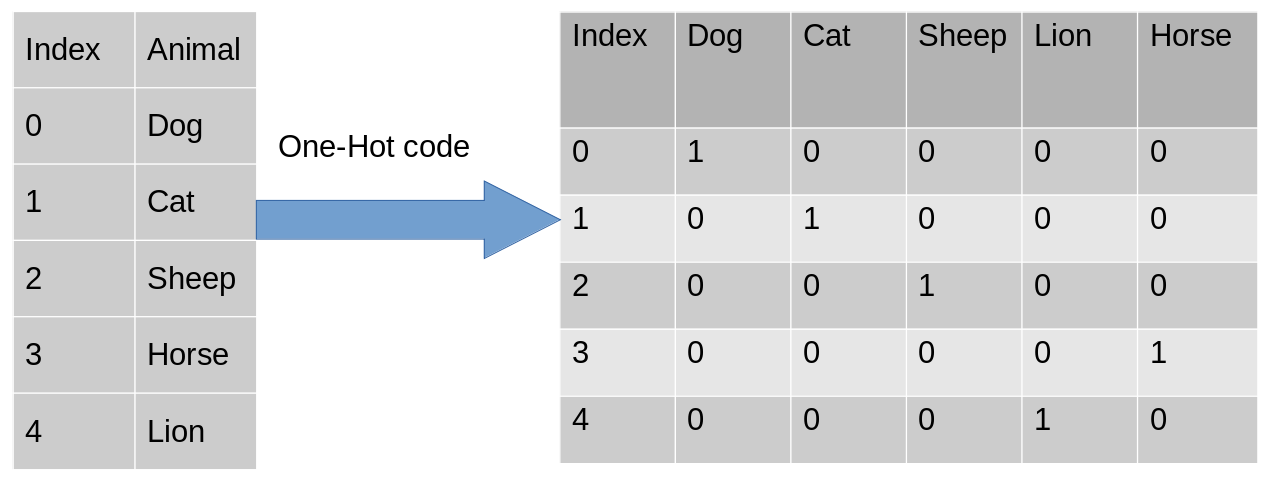After encoding, in the second table, we have dummy variables each representing a category in the feature Animal. Now for each category that is present, we have 1 in the column of that category and 0 for the others. Let’s see how to implement a one-hot encoding in python.

```import category_encoders as ce
import pandas as pd
data=pd.DataFrame({'City':[
]})

#Create object for one-hot encoding
encoder=ce.OneHotEncoder(cols='City',handle_unknown='return_nan',return_df=True,use_cat_names=True)

#Original Data
data
```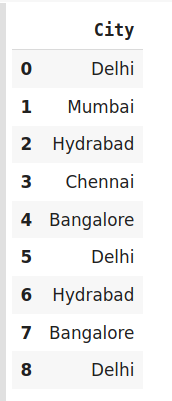```#Fit and transform Data
data_encoded = encoder.fit_transform(data)
data_encoded```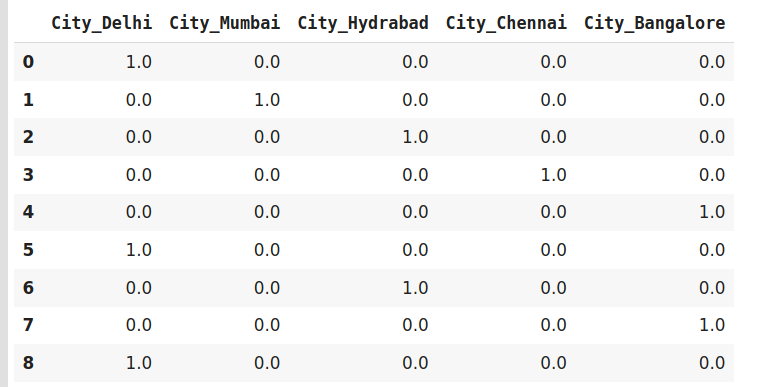Now let’s move to another very interesting and widely used encoding technique i.e Dummy encoding.

## Dummy Encoding

Dummy coding scheme is similar to one-hot encoding. This categorical data encoding method transforms the categorical variable into a set of binary variables (also known as dummy variables). In the case of one-hot encoding, for N categories in a variable, it uses N binary variables. The dummy encoding is a small improvement over one-hot-encoding. Dummy encoding uses N-1 features to represent N labels/categories.

To understand this better let’s see the image below. Here we are coding the same data using both one-hot encoding and dummy encoding techniques. While one-hot uses 3 variables to represent the data whereas dummy encoding uses 2 variables to code 3 categories.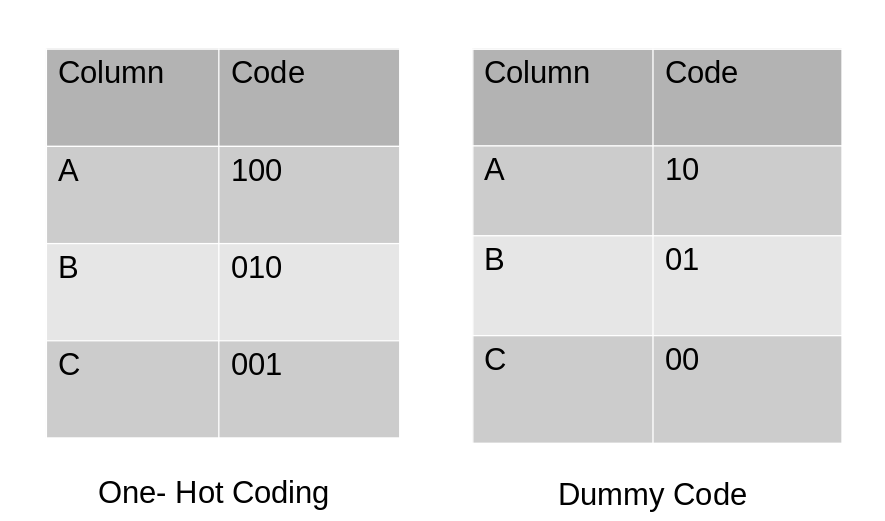Let us implement it in python.

```import category_encoders as ce
import pandas as pd

#Original Data
data```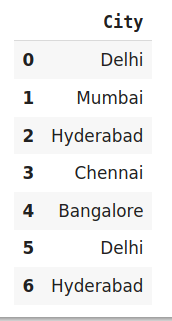```#encode the data
data_encoded=pd.get_dummies(data=data,drop_first=True)
data_encoded```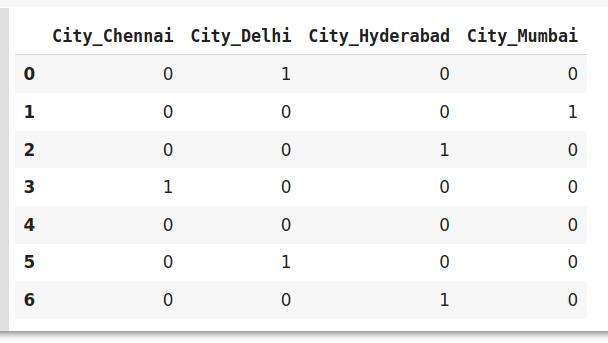Here using drop_first  argument, we are representing the first label Bangalore using 0.

### Drawbacks of  One-Hot and Dummy Encoding

One hot encoder and dummy encoder are two powerful and effective encoding schemes. They are also very popular among the data scientists, But may not be as effective when-

1. A large number of levels are present in data. If there are multiple categories in a feature variable in such a case we need a similar number of dummy variables to encode the data. For example, a column with 30 different values will require 30 new variables for coding.
2. If we have multiple categorical features in the dataset similar situation will occur and again we will end to have several binary features each representing the categorical feature and their multiple categories e.g a dataset having 10 or more categorical columns.

In both the above cases, these two encoding schemes introduce sparsity in the dataset i.e several columns having 0s and a few of them having 1s. In other words, it creates multiple dummy features in the dataset without adding much information.

Also, they might lead to a Dummy variable trap. It is a phenomenon where features are highly correlated. That means using the other variables, we can easily predict the value of a variable.

Due to the massive increase in the dataset, coding slows down the learning of the model along with deteriorating the overall performance that ultimately makes the model computationally expensive. Further, while using tree-based models these encodings are not an optimum choice.

## Effect Encoding:

This encoding technique is also known as Deviation Encoding or Sum Encoding. Effect encoding is almost similar to dummy encoding, with a little difference. In dummy coding, we use 0 and 1 to represent the data but in effect encoding, we use three values i.e. 1,0, and -1.

The row containing only 0s in dummy encoding is encoded as -1 in effect encoding.  In the dummy encoding example, the city Bangalore at index 4  was encoded as 0000. Whereas in effect encoding it is represented by -1-1-1-1.

Let us see how we implement it in python-

```import category_encoders as ce
import pandas as pd

#Original Data
data````encoder.fit_transform(data)`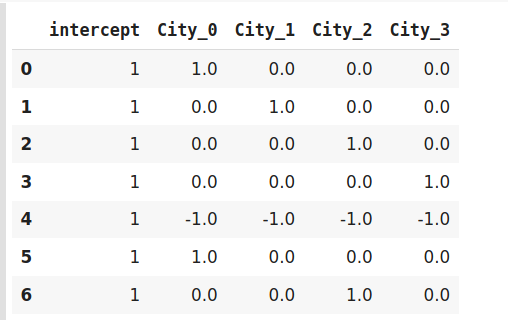Effect encoding is an advanced technique. In case you are interested to know more about effect encoding, refer to this interesting paper.

## Hash Encoder

To understand Hash encoding it is necessary to know about hashing. Hashing is the transformation of arbitrary size input in the form of a fixed-size value. We use hashing algorithms to perform hashing operations i.e to generate the hash value of an input. Further, hashing is a one-way process, in other words, one can not generate original input from the hash representation.

Hashing has several applications like data retrieval, checking data corruption, and in data encryption also. We have multiple hash functions available for example Message Digest (MD, MD2, MD5), Secure Hash Function (SHA0, SHA1, SHA2), and many more.

Just like one-hot encoding, the Hash encoder represents categorical features using the new dimensions. Here, the user can fix the number of dimensions after transformation using n_component argument. Here is what I mean – A feature with 5 categories can be represented using N new features similarly, a feature with 100 categories can also be transformed using N new features. Doesn’t this sound amazing?

By default, the Hashing encoder uses the md5 hashing algorithm but a user can pass any algorithm of his choice. If you want to explore the md5 algorithm, I suggest this paper.

```import category_encoders as ce
import pandas as pd

#Create the dataframe
data=pd.DataFrame({'Month':['January','April','March','April','Februay','June','July','June','September']})

#Create object for hash encoder
encoder=ce.HashingEncoder(cols='Month',n_components=6)
```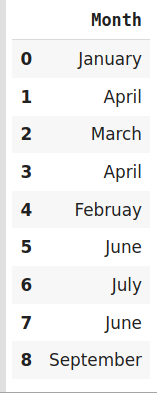```#Fit and Transform Data
encoder.fit_transform(data)```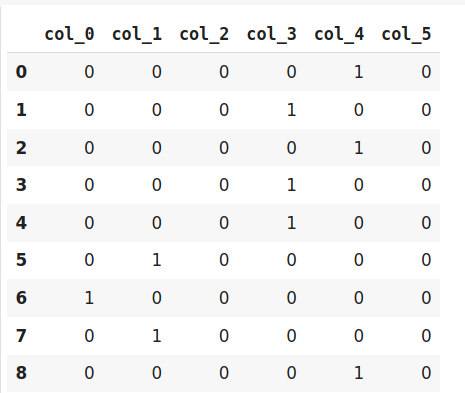Since Hashing transforms the data in lesser dimensions, it may lead to loss of information. Another issue faced by hashing encoder is the collision. Since here, a large number of features are depicted into lesser dimensions, hence multiple values can be represented by the same hash value, this is known as a collision.

Moreover, hashing encoders have been very successful in some Kaggle competitions. It is great to try if the dataset has high cardinality features.

## Binary Encoding

Binary encoding is a combination of Hash encoding and one-hot encoding. In this encoding scheme, the categorical feature is first converted into numerical using an ordinal encoder. Then the numbers are transformed in the binary number. After that binary value is split into different columns.

Binary encoding works really well when there are a high number of categories. For example the cities in a country where a company supplies its products.

```#Import the libraries
import category_encoders as ce
import pandas as pd

#Create the Dataframe

#Create object for binary encoding
encoder= ce.BinaryEncoder(cols=['city'],return_df=True)

#Original Data
data
```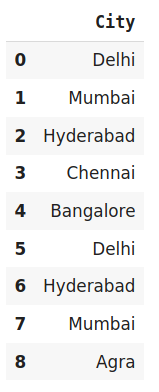```#Fit and Transform Data
data_encoded=encoder.fit_transform(data)
data_encoded```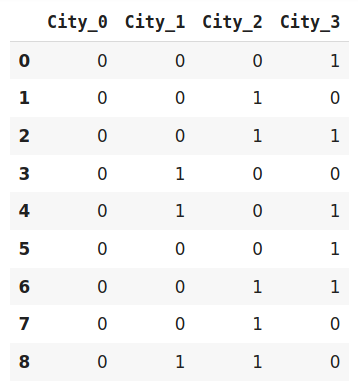Binary encoding is a memory-efficient encoding scheme as it uses fewer features than one-hot encoding. Further, It reduces the curse of dimensionality for data with high cardinality.

## Base N Encoding

Before diving into BaseN encoding let’s first try to understand what is Base here?

In the numeral system, the Base or the radix is the number of digits or a combination of digits and letters used to represent the numbers. The most common base we use in our life is 10  or decimal system as here we use 10 unique digits i.e 0 to 9 to represent all the numbers. Another widely used system is binary i.e. the base is 2. It uses 0 and 1 i.e 2 digits to express all the numbers.

For Binary encoding, the Base is 2 which means it converts the numerical values of a category into its respective Binary form. If you want to change the Base of encoding scheme you may use Base N encoder. In the case when categories are more and binary encoding is not able to handle the dimensionality then we can use a larger base such as 4 or 8.

```#Import the libraries
import category_encoders as ce
import pandas as pd

#Create the dataframe

#Create an object for Base N Encoding
encoder= ce.BaseNEncoder(cols=['city'],return_df=True,base=5)

#Original Data
data``````#Fit and Transform Data
data_encoded=encoder.fit_transform(data)
data_encoded```In the above example, I have used base 5 also known as the Quinary system. It is similar to the example of Binary encoding. While Binary encoding represents the same data by 4 new features the BaseN encoding uses only 3 new variables.

Hence BaseN encoding technique further reduces the number of features required to efficiently represent the data and improving memory usage. The default Base for Base N is 2 which is equivalent to Binary Encoding.

## Target Encoding

Target encoding is a Baysian encoding technique.

Bayesian encoders use information from dependent/target variables to encode the categorical data.

In target encoding, we calculate the mean of the target variable for each category and replace the category variable with the mean value. In the case of the categorical target variables, the posterior probability of the target replaces each category..

```#import the libraries
import pandas as pd
import category_encoders as ce

#Create the Dataframe
data=pd.DataFrame({'class':['A,','B','C','B','C','A','A','A'],'Marks':[50,30,70,80,45,97,80,68]})

#Create target encoding object
encoder=ce.TargetEncoder(cols='class')

#Original Data
Data
```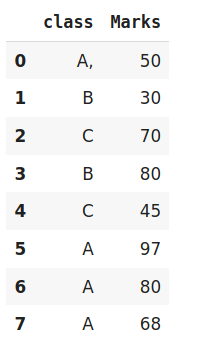```#Fit and Transform Train Data
encoder.fit_transform(data['class'],data['Marks'])```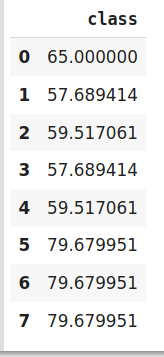We perform Target encoding for train data only and code the test data using results obtained from the training dataset. Although, a very efficient coding system, it has the following issues responsible for deteriorating the model performance-

1. It can lead to target leakage or overfitting. To address overfitting we can use different techniques.
1. In the leave one out encoding, the current target value is reduced from the overall mean of the target to avoid leakage.
2. In another method, we may introduce some Gaussian noise in the target statistics. The value of this noise is hyperparameter to the model.
2. The second issue, we may face is the improper distribution of categories in train and test data. In such a case, the categories may assume extreme values. Therefore the target means for the category are mixed with the marginal mean of the target.

## Endnote

To summarize, encoding categorical data is an unavoidable part of the feature engineering. It is more important to know what coding scheme should we use. Having into consideration the dataset we are working with and the model we are going to use. In this article, we have seen various encoding techniques along with their issues and suitable use cases.

You can also read this article on our Mobile APP